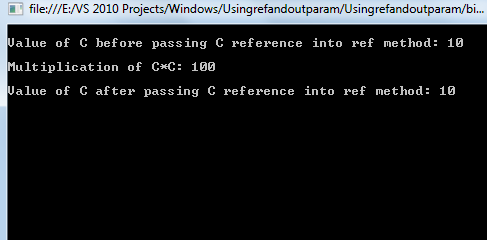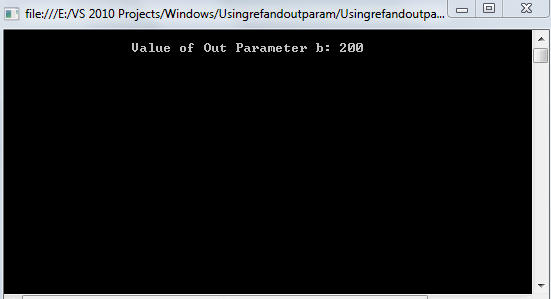Featured

# Ref and Out Parameters in C#

Pass by value is the default behavior of C# methods but we can change that by passing parameters by reference. That does not create any new storage location, so let us start from the basics so beginners can also understand this concept.
What the ref parameter in C# is
The ref keyword is used to pass the parameters to a method by a reference in C#, the parameter passed using the ref keyword does not create a new storage location that is created by a normal value parameter.
When we pass a parameter by reference then the formal argument in the called method becomes an alias to the actual element in the calling method, in other words when you pass the value by reference, you actually pass the address of that value, not a copy of that value.
The following example demonstrates this concept.
```    using System;

namespace Usingrefandoutparam
{
class Program
{
public void Multiplication(ref int a)
{

int d=a* a;
Console.WriteLine("Multiplication of C*C:"+" "+d);

}
static void Main(string[] args)
{
Program p = new Program();
int c = 10;
Console.WriteLine();
Console.WriteLine("Value of C before passing C reference into ref method:"+" "+c);
Console.WriteLine();
p.Multiplication(ref c);
Console.WriteLine();
Console.WriteLine("Value of C after passing C reference into ref method:" + " " + c);
}
}
}
```
In the preceding program we are passing the value to the multiplication method by reference instead of direct value.
Now run the program and see the output.What the Out  parameter in C# is
The out parameter will return the result back to the calling method, similar to the return type but by using the ref parameter the result is passed in an out parameter without creating the new storage location. When a formal parameter is declared as out, then the corresponding actual parameter in the calling method must be declared as out, the out parameter is useful when our method does not return anything and we want something to be get back a result from that method.
Let us see the following example that will demonstrate this concept.

```using System;

namespace Usingrefandoutparam
{
class Program
{
public void Getresult(int a,out int b)
{

b=a+a; //additin result is stored into the b ouput parameter

}

static void Main(string[] args)
{
Program p = new Program();
int Result;
p.Getresult(100, out Result);

Console.WriteLine(Result); //getting the result using Result from b out parameter
}
}
}
```
In the preceding program we declared one out parameter named b in the Getresult method that stores the result of the addition of two numbers and in the calling method we are passing the values and accessing  the result in the Result variable that is stored into the b out variable.
Now run the program, the output will be as follows:Summary